## Screened Poisson Surface Reconstruction (Version 7.0)

links executables usage changes

LINKS
Papers: SGP 2006, ToG 2013
Executables (Win32, Win64)
Source Code GitHub Repository
(Older Versions: V6.13a, V6.13, V6.12, V6.11, V6.1, V6, V5.71, V5.6, V5.5a, V5.1, V5, V4.51, V4.5, V4, V3, V2, V1)

PoissonRecon:
--in <input points>
This string is the name of the file from which the point set will be read.
If the file extension is .ply, the file should be in
PLY format, giving the list of oriented vertices with the x-, y-, and z-coordinates of the positions encoded by the properties x, y, and z and the x-, y-, and z-coordinates of the normals encoded by the properties nx, ny, and nz .
If the file extension is .bnpts, the file should be a binary file, consisting of blocks of 6 32-bit floats: x-, y-, and z-coordinates of the point's position, followed by the x-, y-, and z-coordinates of the point's normal. (No information about the number of oriented point samples should be specified.)
Otherwise, the file should be an ascii file with groups of 6, white space delimited, numbers: x-, y-, and z-coordinates of the point's position, followed by the x-, y- and z-coordinates of the point's normal. (No information about the number of oriented point samples should be specified.)
[--out <output triangle mesh>]
This string is the name of the file to which the triangle mesh will be written. The file is written in PLY format.
[--color <pull factor>]
If specified, the reconstruction code assumes that the input is equipped with colors and will extrapolate the color values to the vertices of the reconstructed mesh. The floating point value specifies the relative importance of finer color estimates over lower ones. (In practice, we have found that a pull factor of 16 works well.)
[--voxel <output voxel grid>]
This string is the name of the file to which the sampled implicit function will be written. The filw is wrtten out in binary, with the first 4 bytes corresponding to the (integer) sampling resolution, 2^d, and the next 4 x 2^d x 2^d x 2^d bytes corresponding to the (single precision) floating point values of the implicit function.
[--depth <reconstruction depth>]
This integer is the maximum depth of the tree that will be used for surface reconstruction. Running at depth d corresponds to solving on a voxel grid whose resolution is no larger than 2^d x 2^d x 2^d. Note that since the reconstructor adapts the octree to the sampling density, the specified reconstruction depth is only an upper bound.
The default value for this parameter is 8.
[--fullDepth <adaptive octree depth>]
This integer specifies the depth beyond depth the octree will be adapted. At coarser depths, the octree will be complete, containing all 2^d x 2^d x 2^d nodes.
The default value for this parameter is 5.
[--voxelDepth <voxel sampling depth>]
This integer is the depth of the regular grid over which the implicit function is to be sampled. Running at depth d corresponds to sampling on a voxel grid whose resolution is 2^d x 2^d x 2^d.
The default value for this parameter is the value of the --depth parameter.
[--cgDepth <conjugate gradients solver depth>]
This integer is the depth up to which a conjugate-gradients solver will be used to solve the linear system. Beyond this depth Gauss-Seidel relaxation will be used.
The default value for this parameter is 0.
[--scale <scale factor>]
This floating point value specifies the ratio between the diameter of the cube used for reconstruction and the diameter of the samples' bounding cube.
The default value is 1.1.
[--samplesPerNode <minimum number of samples>]
This floating point value specifies the minimum number of sample points that should fall within an octree node as the octree construction is adapted to sampling density. For noise-free samples, small values in the range [1.0 - 5.0] can be used. For more noisy samples, larger values in the range [15.0 - 20.0] may be needed to provide a smoother, noise-reduced, reconstruction.
The default value is 1.0.
[--pointWeight <interpolation weight>]
This floating point value specifies the importants that interpolation of the point samples is given in the formulation of the screened Poisson equation.
The results of the original (unscreened) Poisson Reconstruction can be obtained by setting this value to 0.
The default value for this parameter is 4.
[--iters <GS iters>]
This integer value specifies the number of Gauss-Seidel relaxations to be performed at each level of the hiearchy.
The default value for this parameter is 8.
[--threads <number of processing threads>]
This integer specifies the number of threads across which the reconstruction algorithm should be parallelized.
The default value for this parameter is equal to the numer of (virtual) processors on the executing machine.
[--confidence]
Enabling this flag tells the reconstructor to use the size of the normals as confidence information. When the flag is not enabled, all normals are normalized to have unit-length prior to reconstruction.
[--nWeights]
Enabling this flag tells the reconstructor to use the size of the normals to modulate the interpolation weights. When the flag is not enabled, all points are given the same weight.
[--polygonMesh]
Enabling this flag tells the reconstructor to output a polygon mesh (rather than triangulating the results of Marching Cubes).
[--density]
Enabling this flag tells the reconstructor to output the estimated depth values of the iso-surface vertices.
[--verbose]
Enabling this flag provides a more verbose description of the running times and memory usages of individual components of the surface reconstructor.
SurfaceTrimmer:
--in <input triangle mesh>
This string is the name of the file from which the triangle mesh will be read. The file is read in PLY format and it is assumed that the vertices have a value field which stores the signal's value. (When run with --density flag, the reconstructor will output this field with the mesh vertices.)
--trim <trimming value>
This floating point values specifies the value for mesh trimming. The subset of the mesh with signal value less than the trim value is discarded.
[--out <output triangle mesh>]
This string is the name of the file to which the triangle mesh will be written. The file is written in PLY format.
[--smooth <smoothing iterations>]
This integer values the number of umbrella smoothing operations to perform on the signal before trimming.
The default value is 5.
[--aRatio <island area ratio>]
This floating point value specifies the area ratio that defines a disconnected component as an "island". Connected components whose area, relative to the total area of the mesh, are smaller than this value will be merged into the output surface to close small holes, and will be discarded from the output surface to remove small disconnected components.
The default value 0.001.
[--polygonMesh]
Enabling this flag tells the trimmer to output a polygon mesh (rather than triangulating the trimming results).

USAGE
For testing purposes, three point sets are provided:
1. Eagle: A set of 796,825 oriented point samples with color (represented in PLY format) was obtained in the EPFL Scanning 3D Statues from Photos course.
• The original Poisson Reconstruction algorithm can be invoked by calling:
`% PoissonRecon --in eagle.points.ply --out eagle.unscreened.ply --depth 10 --pointWeight 0`
using the --pointWeight 0 argument to disable the screening.
• By default, screening is enabled so the call:
`% PoissonRecon --in eagle.points.ply --out eagle.screened.ply --depth 10`
produces a reconstruction that more faithfully fits the input point positions.
• A reconstruction of the eagle that extrapolates the color values from the input samples can be obtained by calling:
`% PoissonRecon --in eagle.points.ply --out eagle.screened.color.ply --depth 10 --color 16`
using the --color 16 to indicate both that color should be used, and the extent to which finer color estimates should be preferenced over coarser estimates.
• Finally, a reconstruction the eagle that does not close up the holes can be obtained by first calling:
`% PoissonRecon --in eagle.points.ply --out eagle.screened.color.ply --depth 10 --color 16 --density`
using the --density flag to indicate that density estimates should be output with the vertices of the mesh, and then calling:
`% SurfaceTrimmer --in eagle.screened.color.ply --out eagle.screened.color.trimmed.ply --trim 7`
to remove all subsets of the surface where the sampling density corresponds to a depth smaller than 7.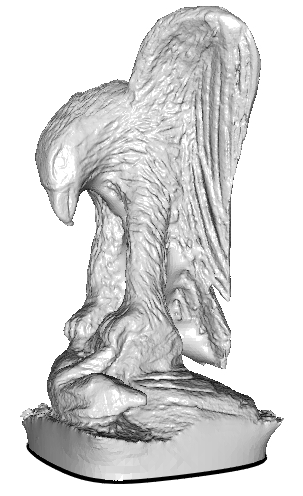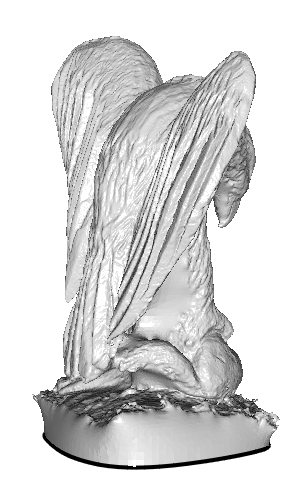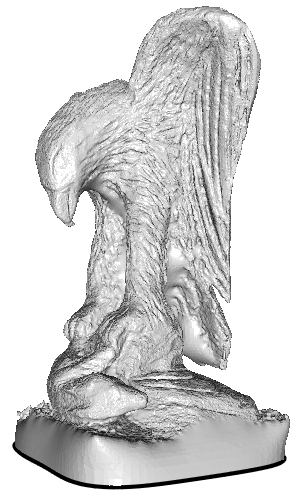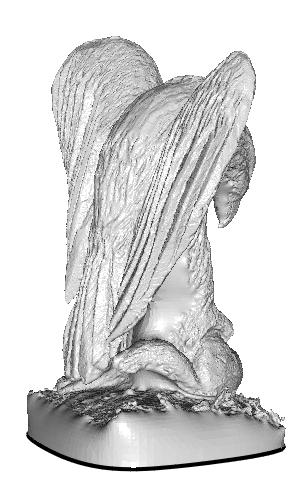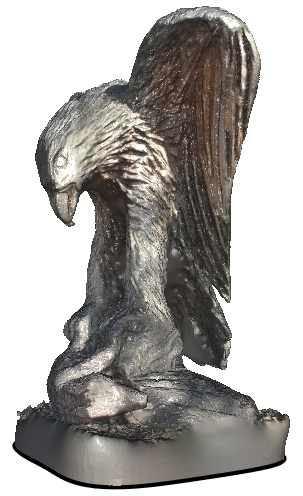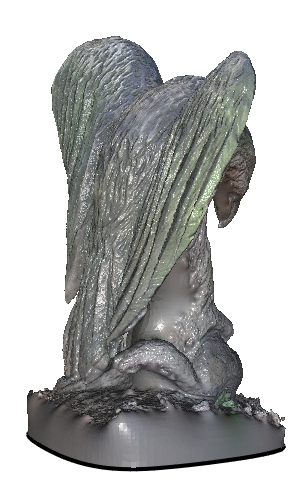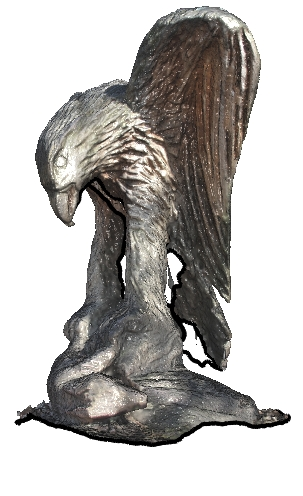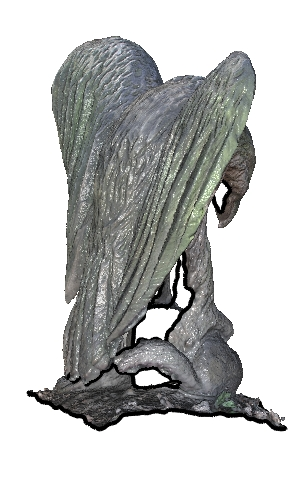Unscreened Screened Screened + Color Screened + Color + Trimmed
2. Bunny: A set of 362,271 oriented point samples (represented in PLY format) was obtained by merging the data from the original Stanford Bunny range scans. The orientation of the sample points was estimated using the connectivity information within individual range scans.
The surface of the model can be reconstructed by calling the surface reconstructor as follows:
`% PoissonRecon --in bunny.points.ply --out bunny.ply --depth 10`
3. Horse: A set of 100,000 oriented point samples (represented in ASCII format) was obtained by sampling a virtual horse model with a sampling density proportional to curvature, giving a set of non-uniformly distributed points.
The surface of the model can be reconstructed by calling the surface reconstructor as follows:
`% PoissonRecon --in horse.npts --out horse.ply --depth 10`
To convert the binary PLY format to Hugues Hoppe's ASCII mesh format, a Perl script is provided.
As an examples, the reconstructed bunny can be converted into the ASCII mesh format as follows:
`% ply2mesh.pl bunny.ply > bunny.m`

CHANGES
Version 3:
1. The implementation of the --samplesPerNode parameter has been modified so that a value of "1" more closely corresponds to a distribution with one sample per leaf node.
2. The code has been modified to support compilation under MSVC 2010 and the associated solution and project files are now provided. (Due to a bug in the Visual Studios compiler, this required modifying the implementation of some of the bit-shifting operators.)
Version 4:
1. The code supports screened reconstruction, with interpolation weight specified through the --pointWeight parameter.
2. The code has been implemented to support parallel processing, with the number of threads used for parallelization specified by the --threads parameter.
3. The input point set can now also be in PLY format, and the file-type is determined by the extension, so that the --binary flag is now obsolete.
4. At depths coarser than the one specified by the value --minDepth the octree is no longer adaptive but rather complete, simplifying the prolongation operator.
Version 4.5:
1. The algorithmic complexity of the solver was reduced from log-linear to linear.
Version 4.51:
1. Smart pointers were added to ensure that memory accesses were in bounds.
Version 5:
1. The --density flag was added to the reconstructor to output the estimated depth of the iso-vertices.
2. The SurfaceTrimmer executable was added to support trimming off the subset of the reconstructed surface that are far away from the input samples, thereby allowing for the generation of non-water-tight surface.
Version 5.1:
1. Minor bug-fix to address incorrect neighborhood estimation in the octree finalization.
Version 5.5a:
1. Modified to support depths greater than 14. (Should work up to 18 or 19 now.)
2. Improved speed and memory performance by removing the construction of integral and value tables.
3. Fixed a bug in Version 5.5 that used memory and took more time without doing anything useful.
Version 5.6:
1. Added the --normalWeight flag to support setting a point's interpolation weight in proportion to the magnitude of its normal.
Version 5.7:
1. Modified the setting of the constraints, replacing the map/reduce implementation with OpenMP atomics to reduce memory usage.
2. Fixed bugs that caused numerical overflow when processing large point clouds on multi-core machines.
3. Improved efficiency of the iso-surface extraction phse.
Version 5.71:
1. Added the function GetSolutionValue to support the evaluation of the implicit function at a specific point.
Version 6:
1. Modified the solver to use Gauss-Seidel relaxation instead of conjugate-gradients at finer resolution.
2. Re-ordered the implementation of the solver so that only a windowed subset of the matrix is in memory at any time, thereby reducing the memory usage during the solver phase.
3. Separated the storage of the data associated with the octree nodes from the topology.
Version 6.1:
1. Re-ordered the implementation of the iso-surface extraction so that only a windowed subset of the octree is in memory at any time, thereby reducing the memory usage during the extracted phase.
Version 6.11:
1. Fixed a bug that created a crash in the evaluation phase when --pointWeight is set zero.
Version 6.12:
1. Removed the OpenMP firstprivate directive as it seemed to cause trouble under Linux compilations.
Version 6.13:
1. Added a MemoryPointStream class in PointStream.inl to support in-memory point clouds.
2. Modified the signature of Octree::SetTree in MultiGridOctreeData.h to take in a pointer to an object of type PointStream rather than a file-name.
Version 6.13a:
1. Modified the signature of Octree::SetIsoSurface to rerun a void. [cloudcompare]
2. Added a definition of SetIsoVertexValue supporting double precision vertices. [cloudcompare]
3. Removed Time.[h/cpp] from the repository. [cloudcompare/asmaloney]
4. Fixed assignment bug in Octree::SetSliceIsoVertices. [asmaloney]
5. Fixed initialization bug in SortedTreeNodes::SliceTableData and SortedTreeNodes::XSliceTableData. [asmaloney]
6. Included stdlib.h in Geometry.h. [asmaloney]
7. Fixed default value bug in declaration of Octree::SetTree. [asmaloney]
Version 7.0:
1. Added functionality to support color extrapolation if present in the input.
2. Modified a bug with the way in which sample contributions were scaled.

HOME